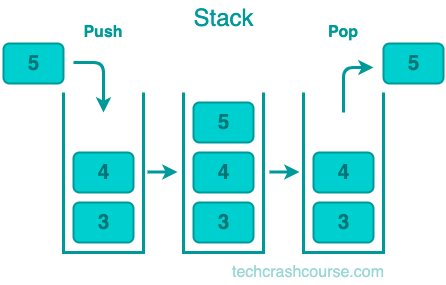# Stack Data Structure

A Stack is a linear data structure that follows the Last In First Out (LIFO) principle. It means that the last item added to the stack will be the first item to be removed. A stack is like a pile of books. When you add a new book to the pile, it becomes the top of the stack. When you remove a book from the pile, you remove the top one first.

A stack is a collection of elements that supports two operations: push and pop. The push operation adds an element to the top of the stack, and the pop operation removes the top element from the stack.## Representation of Stack

A stack can be represented using an array or a linked list.

### Array Representation of Stack

In the array representation of a stack, we use an array to store the elements of the stack. We also need a variable to keep track of the top of the stack. The top of the stack is the index of the last element added to the stack.

### Linked List Representation of Stack

In the linked list representation of a stack, we use a linked list to store the elements of the stack. Each node in the linked list represents an element of the stack, and the top of the stack is the head of the linked list.

## Uses of Stack Data Structure

• Function call stack: When a function is called, its return address and parameters are pushed onto the stack. When the function returns, these values are popped off the stack.
• Expression evaluation: In computer science, expressions are usually evaluated using a stack. For example, to evaluate the expression 2 * (3 + 4), we push 2 onto the stack, then push 3 and 4 onto the stack, then pop 3 and 4 off the stack and add them together, then multiply the result by 2.
• Backtracking: Stacks can be used to implement backtracking algorithms. In a backtracking algorithm, we keep track of the choices we have made so far on the stack. If we reach a dead end, we pop the choices off the stack until we find a choice that leads to a solution.
• Undo/Redo operations: Stacks can be used to implement undo and redo operations in software applications. Each operation is pushed onto the stack, and undoing an operation involves popping it off the stack.

### Advantages of Stack Data Structure

• They are easy to implement and use.

• They have a simple and intuitive interface.

• They are efficient for adding and removing elements from the top of the stack.

### Disadvantages of Stack Data Structure

• They have limited functionality, as they only support push and pop operations.

• They can only be used to access elements in LIFO order.

## Common Stack Operations

• Push: Push operation inserts an element onto the top of the stack. It takes a single parameter, the value to be pushed onto the stack, and increases the stack pointer to accommodate the new element.

• Pop: Pop operation removes the top element from the stack. It returns the value of the element that was popped and decreases the stack pointer.

• Peek: Peek operation returns the value of the top element from the stack without removing it.

• isEmpty: isEmpty operation returns a Boolean value indicating whether the stack is empty or not.

• isFull: isFull operation returns a Boolean value indicating whether the stack is full or not.

• Size: Size operation returns the number of elements currently in the stack.

## Stack Data Structure Implementation Program

Here's a simple implementation of the stack data structure in C.

```#include <stdio.h>
#include <stdlib.h>

#define MAX_SIZE 10

int stack[MAX_SIZE];
int top = -1;

void push(int value) {
if (top == MAX_SIZE - 1) {
printf("Stack overflow\n");
return;
}
stack[++top] = value;
}

int pop() {
if (top == -1) {
printf("Stack underflow\n");
return -1;
}
return stack[top--];
}

int peek() {
if (top == -1) {
printf("Stack is empty\n");
return -1;
}
return stack[top];
}

int isEmpty() {
}

int isFull() {
}

int size() {
}

int main() {
push(1);
push(2);
push(3);

printf("Stack size is %d\n", size());
printf("Top element is %d\n", peek());
printf("Popped element is %d\n", pop());
printf("Top element is %d\n", peek());
printf("Stack is%s empty\n", isEmpty() ? "" : " not");
printf("Stack is%s full\n", isFull() ? "" : " not");

return 0;
}
```
Output
```Stack size is 3
Top element is 3
Popped element is 3
Top element is 2
Stack is not empty
Stack is not full
```

In this program, we define an array stack and an integer variable top to keep track of the top element of the stack. The push function adds an element to the top of the stack, while pop removes the top element from the stack. The peek function returns the value of the top element without removing it. The isEmpty function checks if the stack is empty and returns a Boolean value, and the isFull function checks if the stack is full and returns a Boolean value. Finally, the size function returns the number of elements currently in the stack.

We then demonstrate the use of these operations in the main function by pushing three elements onto the stack, printing the stack size, the value of the top element, and then popping an element from the stack. We print the value of the top element again and check if the stack is empty or not.